| my account | login-logout | resources | support | catalog | home | get webcard |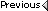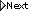» Online Classroom   » Inland and Coastal Navigation   » Public Discussion of Inland and Coastal Navigation   » Notes on Dead Reckoning: Solving for longitude by calculator

Author Topic: Notes on Dead Reckoning: Solving for longitude by calculator
 BCraig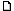posted January 08, 2008 03:23 PM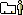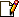Question 1. When calculating latitude from given (i)time run, (ii)speed and (iii)course with a calculator the formula is given at p. 4 of 'Notes on dead reckoning" as Lat=Distance*Cos(Course). In contrast the longitude formula is given as Lo=Dist*Sin(course)/Cos(Lat). Why is it necessary to divide by Cos(Lat)? Question 2. In the example on p. 4 of "Notes on Dead Reckoning" the Cosine of 26 is used. Where does 26 come from? From: US
 David Burchposted January 11, 2008 11:05 PMI apologize for the delay. we must have all missed the mail announcement that your post was here. i will try to get to this tonight or if not tomorrow. in the future if you do not find an answer online in 48 hr do not heistate to send an email to [email protected] (which immediately returns the right email to use for the question) or call 800-955-8328.also if you are signed up for the online course, it is more efficient to post in the student discussion forum as more instructors are watching that one. --david From: Starpath, Seattle, WA
 David Burchposted January 11, 2008 11:16 PMthe reason for this is the way the mercator projection works. the distance between longitude lines gets smaller as you go north, like sections of an organge peel. That decrease with latitude can be accounted for simply by reducing the size of the interval by a factor of cos (lat). thus we can say the number of nautical miles (D) per 1° of longitude is given byD = 60 x cos (lat).at the equator cos 0 = 1 so there are 60 nmi per 1° of longitude, but in seattle at 47 N, there are 60 x cos (47) = 60 x 0.68199836 = 40.92 nmiby the way, did you know you can type 60 x cos(47 degrees) into the google search engine and get out 40.9199016==========Part 2. The 26° is the DR lat rounded off to the nearest degree in the example being worked (see the top lines of page 3)... which in that example is being worked with Traverse Tables, not by the formula. ==========again, i apologize for the delaydoes that answer the question. From: Starpath, Seattle, WA
 BCraigposted January 29, 2008 12:54 PMUsing a calculator only (example 3 of the dead reckoning practice problems) how would I know what latitude to plug into the formula? The example in the worksheet is 26° which is the DR lat rounded up to the nearest degree -- shouldn't it be 25? From: US
 David Burchposted January 30, 2008 02:00 PMThe initial DR lat in the problem is 25° 36.5'. in the simplest solution you round this to the nearest degree which would be 26.Or since you are using a calculator, you could use 25.5 or since 36.5/60 = .608 you could use 25.608.BUT... to do the best with this type of compuation, it is best to use the "mid-latitude" ie, find dLat first, then you have lat 2, ie lat1+dLat. Then take (lat2+lat1)/2 = mid lat and use that in the cosine term. From: Starpath, Seattle, WA
 michaelcamposted April 05, 2010 10:11 AMIs this topic in the Inland and Coastal Navigation book?? If so where? Thanks. From: Columbia, MD
 David Burchposted April 05, 2010 10:39 AMCalculator solutions to DR is not in the textbook, but if you post this question in the online course we can post there an article on the subject. --david From: Starpath, Seattle, WA

 All times are Pacific